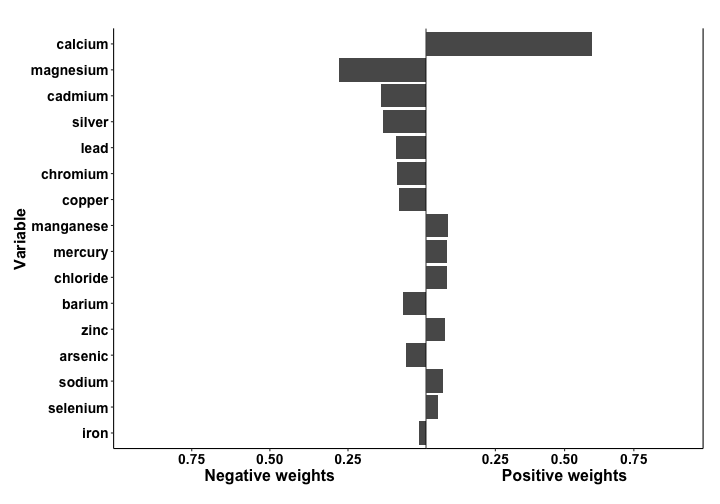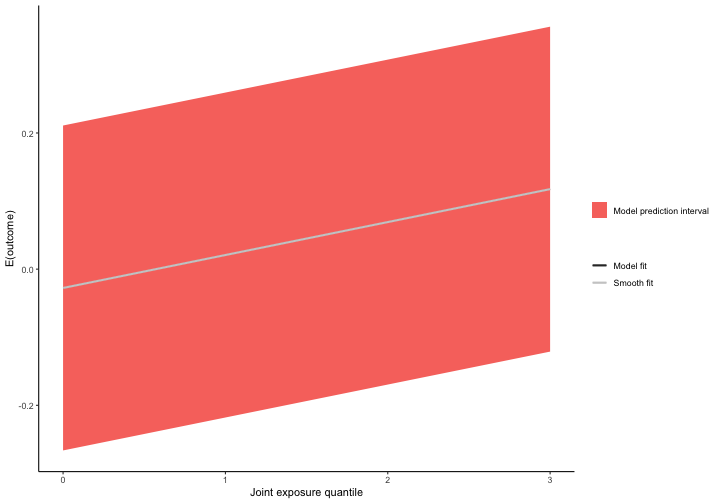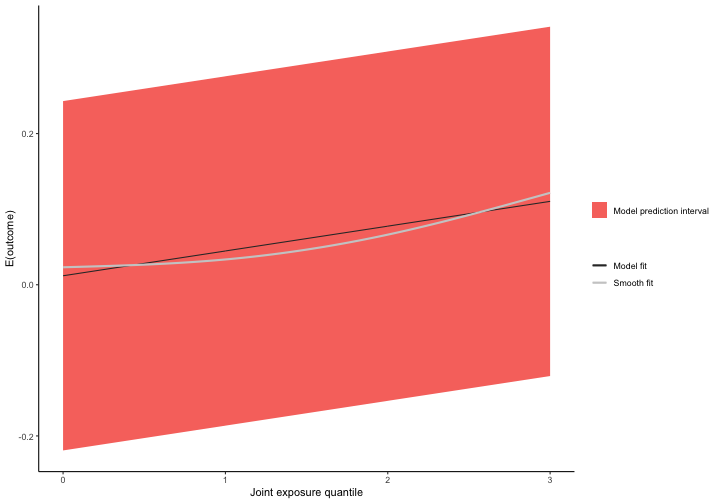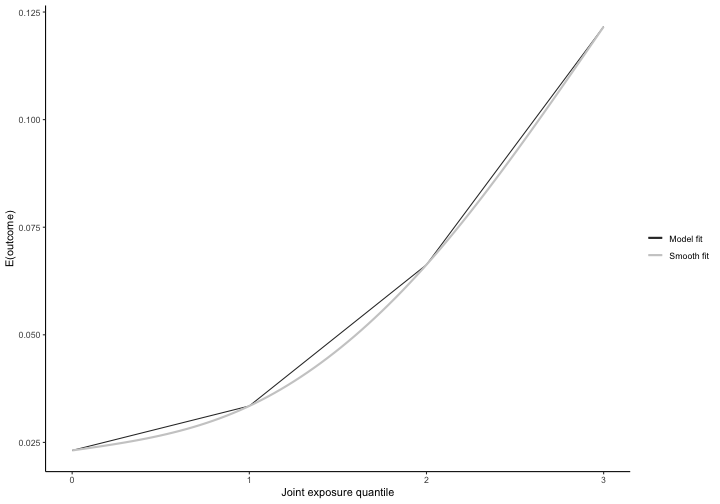## Introduction

`qgcomp` is a package to implement g-computation for analyzing the effects of exposure mixtures. Quantile g-computation yields estimates of the effect of increasing all exposures by one quantile, simultaneously. This, it estimates a “mixture effect” useful in the study of exposure mixtures such as air pollution, diet, and water contamination.

The implementation in `qgcomp` is based on a generalization of weighted quantile sums (WQS) regression, which estimates the expected change in an outcome under a hypothetical intervention to increase all exposures in the mixture by one quantile. In the case in which all exposures have linear, additive effects on the quantile scale (i.e. after transforming all exposures into categorical variables defined by quantiles) `qgcomp` and WQS regression are asymptotically equivalent when all variables have effects in the same direction (the 'directional homogeneity' assumption of WQS). In moderate samples when underlying assumptions are not met exactly, WQS will tend to yield more biased effect estimates due to the assumption of directional homogeneity, and may also have lower precision due to the use of sample splitting. In cases in which the assumptions underlying WQS are met,`qgcomp` provides valid estimates of WQS 'weights', which estimate the relative contribution of each exposure to the overall mixture effect. Thus, `qgcomp` will provide valid effect estimates of the entire exposure mixture in general cases, while also allowing deviations from linearity and additivity assumptions.

Using terminology from methods developed for causal effect estimation, quantile g-computation estimates the parameters of a marginal structural model that characterizes the change in the expected potential outcome given a joint intervention on all exposures, possibly conditional on confounders. Under the assumptions of exchangeability, causal consistency, positivity, no interference, and correct model specification, this model yields a causal effect for an intervention on the mixture as a whole. While these assumptions may not be met exactly, they provide a useful roadmap for how to interpret the results of a qgcomp fit, and where efforts should be spent in terms of ensuring accurate model specification and selection of exposures that are sufficient to control co-pollutant confounding.

## How to use the `qgcomp` package

Here we use a running example from the `metals` dataset from the from the package `qgcomp` to demonstrate some features of the package and method.

Similar examples can be seen in the `gQWS` package help files, which implements WQS regression.

``````library(qgcomp)
library(knitr)
data("metals", package="qgcomp")
``````
``````##      arsenic     barium     cadmium    calcium    chloride    chromium
## 1  0.3455400  0.3045971 -0.97805683  0.1945599 -0.28741380 -0.01895504
## 2  0.3524465  0.7339532  0.15698285 -0.4840742 -0.22475166 -0.05782744
## 3 -0.8944167 -0.4928027 -0.70327450 -0.6740917 -0.26631784 -0.01848287
## 4 -0.9563741  0.3850867  0.19118004 -0.8098185 -0.21337264 -0.08168334
## 5  0.6837788  0.3090797  0.58266451  0.6560310  0.01740394 -0.07223622
## 6 -0.4443633  0.6887725  0.02339795 -0.1854752 -0.08854167 -0.07149450
##        copper       iron         lead   magnesium  manganese    mercury
## 1 -0.54902626 -0.2007045  0.056168900 -0.50446272 -0.5046921  1.5270339
## 2  0.18406536 -0.2594449  0.004757928  0.07135149 -0.3694587 -1.4132400
## 3 -0.36616139 -0.2536885 -0.159633376  0.07135149 -0.4680459 -1.5434921
## 4  0.23675057 -0.2507978 -0.040773337 -0.50446272 -0.2606127  0.5489630
## 5  0.08198291 -0.2354496 -0.185916726  3.91011288 -0.4622545  0.5607343
## 6 -0.32018871 -0.2637024 -0.005194064  1.41491798 -0.2841611 -1.3850396
##      nitrate     nitrite          ph   selenium     silver      sodium
## 1 -0.5947917 -0.08703449  0.60728873 -0.6248274  0.4311889 -0.42323080
## 2 -0.4822203 -0.82868159  0.10831329  0.4254736 -0.9077198 -0.40641442
## 3 -0.6295622  0.87304583  0.27463843 -0.6288835  1.3919820 -0.40641442
## 4  0.9921658 -1.36311161 -0.88963760 -0.6163625  0.6048928 -0.38455312
## 5  2.9272013  0.29939869 -0.22433701  0.3193482 -0.5209047 -0.09867466
## 6 -0.1421493 -0.99400679 -0.05801186 -0.3745180 -0.5329035 -0.30047122
##       sulfate total_alkalinity total_hardness       zinc mage35
## 1 -0.15947022       -0.3066230     0.04820906 -0.2325039      1
## 2 -0.10898731       -0.8242949    -0.45714647 -0.1550943      1
## 3 -0.19430715       -1.0136871    -0.65310065 -0.0605004      0
## 4 -0.19457399       -1.3672191    -0.89030835 -0.1925551      0
## 5  0.04400840        1.3347758     1.49208199 -0.1754426      1
## 6 -0.05639503       -0.2687446     0.15134284 -0.1925881      0
##               y disease_state
## 1  0.1148135326             0
## 2 -0.0703945843             0
## 3 -0.0004721879             0
## 4  0.0481693629             0
## 5  0.2295161743             1
## 6  0.0397177515             1
``````

### Example 1: linear model

``````# we save the names of the mixture variables in the variable "Xnm"
Xnm <- c(
'sodium','zinc'
)
covars = c('nitrate','nitrite','sulfate','ph', 'total_alkalinity','total_hardness')

# Example 1: linear model
# Run the model and save the results "qc.fit"
system.time(qc.fit <- qgcomp.noboot(y~.,dat=metals[,c(Xnm, 'y')], family=gaussian()))
``````
``````## Including all model terms as exposures of interest
``````
``````##    user  system elapsed
##   0.019   0.002   0.020
``````
``````#   user  system elapsed
#  0.011   0.002   0.018

# contrasting other methods with computational speed
# WQS regression
#system.time(wqs.fit <- gwqs(y~NULL,mix_name=Xnm, data=metals[,c(Xnm, 'y')], family="gaussian"))
#   user  system elapsed
# 35.775   0.124  36.114

# Bayesian kernel machine regression (note that the number of iterations here would
#  need to be >5,000, at minimum, so this underestimates the run time by a factor
#  of 50+
#system.time(bkmr.fit <- kmbayes(y=metals\$y, Z=metals[,Xnm], family="gaussian", iter=100))
#   user  system elapsed
# 81.644   4.194  86.520

#first note that qgcomp is very fast

# View results: scaled coefficients/weights and statistical inference about
# mixture effect
qc.fit
``````
``````## Scaled effect size (positive direction, sum of positive coefficients = 0.118)
##   calcium manganese   mercury  chloride      zinc    sodium  selenium
##    0.5974    0.0791    0.0761    0.0739    0.0704    0.0658    0.0373
##
## Scaled effect size (negative direction, sum of negative coefficients = -0.0732)
##    0.2673    0.1758    0.1303    0.1047    0.0872    0.0831    0.0796
##    barium      iron
##    0.0584    0.0136
##
## Mixture slope parameters (Delta method CI):
##
##      Estimate Std. Error  Lower CI Upper CI t value Pr(>|t|)
## psi1 0.044589   0.019180 0.0069964 0.082182  2.3247  0.02055
``````

One advantage of quantile g-computation over other methods that estimate “mixture effects” (the effect of changing all exposures at once), is that it is very computationally efficient. Contrasting methods such as WQS (`gWQS` package) and Bayesian Kernel Machine regression (`bkmr` package), quantile g-computation can provide results many orders of magnitude faster. For example, the example above ran 3000X faster for quantile g-computation versus WQS regression, and we estimate the speedup would be several hundred thousand times versus Bayesian kernel machine regression.

Quantile g-computation yields fixed weights in the estimation procedure, similar to WQS regression. However, note that the weights from `qgcomp.noboot` can be negative or positive. When all effects are linear and in the same direction (“directional homogeneity”), quantile g-computation is equivalent to weighted quantile sum regression in large samples.

The overall mixture effect from quatile g-computation (psi1) is interpreted as the effect on the outcome of increasing every exposure by one quantile, possibly conditional on covariates. Given the overall exposure effect, the weights are considered fixed and so

### Example 2: conditional odds ratio, marginal odds ratio in a logistic model

This example introduces the use of a binary outcome in `qgcomp` via the `qgcomp.noboot` function, which yields a conditional odds ratio or the `qgcomp.boot`, which yields a marginal odds ratio or risk/prevalence ratio. These will not equal each other when there are non-exposure covariates (e.g. confounders) included in the model because the odds ratio is not collapsible (both are still valid). Marginal parameters will yield estimates of the population average exposure effect, which is often of more interest due to better interpretability over conditional odds ratios. Further, odds ratios are not generally of interest when risk ratios can be validly estimated, so `qgcomp.boot` will estimate the risk ratio by default for binary data (set rr=FALSE to allow estimation of ORs when using `qgcomp.boot`).

``````qc.fit2 <- qgcomp.noboot(disease_state~., expnms=Xnm,
data = metals[,c(Xnm, 'disease_state')], family=binomial(),
q=4)
qcboot.fit2 <- qgcomp.boot(disease_state~., expnms=Xnm,
data = metals[,c(Xnm, 'disease_state')], family=binomial(),
q=4, B=10,# B should be 200-500+ in practice
seed=125, rr=FALSE)
qcboot.fit2b <- qgcomp.boot(disease_state~., expnms=Xnm,
data = metals[,c(Xnm, 'disease_state')], family=binomial(),
q=4, B=10,# B should be 200-500+ in practice
seed=125, rr=TRUE)

# Compare a qgcomp.noboot fit:
qc.fit2
``````
``````## Scaled effect size (positive direction, sum of positive coefficients = 1.83)
##  mercury  arsenic  cadmium selenium   silver   copper chloride   sodium
##  0.41512  0.22194  0.11081  0.11037  0.03762  0.03140  0.02883  0.02714
## chromium   barium
##  0.01515  0.00161
##
## Scaled effect size (negative direction, sum of negative coefficients = -0.851)
## manganese      lead      zinc      iron magnesium   calcium
##    0.5635    0.2145    0.1342    0.0358    0.0307    0.0213
##
## Mixture log(OR) (Delta method CI):
##
##      Estimate Std. Error Lower CI Upper CI Z value Pr(>|z|)
## psi1  0.97669    0.37724   0.2373   1.7161   2.589 0.009625
``````
``````# and a qgcomp.boot fit:
qcboot.fit2
``````
``````## Mixture log(OR) (bootstrap CI):
##
##      Estimate Std. Error Lower CI Upper CI Z value Pr(>|z|)
## psi1  0.97669    0.30828  0.37247   1.5809  3.1682 0.001534
``````
``````# and a qgcomp.boot fit, where the risk/prevalence ratio is estimated,
#  rather than the odds ratio:
qcboot.fit2b
``````
``````## Mixture log(RR) (bootstrap CI):
##
##      Estimate Std. Error Lower CI Upper CI Z value  Pr(>|z|)
## psi1  0.31318    0.07747  0.16134  0.46502  4.0426 5.286e-05
``````

### Example 3: adjusting for covariates, plotting estimates

In the following code we run a maternal age-adjusted linear model with `qgcomp` (`family = "gaussian"`).

``````qc.fit3 <- qgcomp.noboot(y ~ mage35 + arsenic + barium + cadmium + calcium + chloride +
chromium + copper + iron + lead + magnesium + manganese +
mercury + selenium + silver + sodium + zinc,
expnms=Xnm,
metals, family=gaussian(), q=4)
plot(qc.fit3)
qcboot.fit3 <- qgcomp.boot(y ~ mage35 + arsenic + barium + cadmium + calcium + chloride +
chromium + copper + iron + lead + magnesium + manganese +
mercury + selenium + silver + sodium + zinc,
expnms=Xnm,
metals, family=gaussian(), q=4, B=10,# B should be 200-500+ in practice
seed=125)
plot(qcboot.fit3)
``````From the first plot we see weights from `qgcomp.noboot` function, which include both positive and negative effect directions. Using `qgcomp.boot` also allows us to assess linearity of the total exposure effect (the second plot). Similar output is available for WQS (`gWQS` package), though WQS results will generally be less interpretable when exposure effects are non-linear (see below how to do this with `qgcomp.boot`.

The plot for the `qcboot.fit3` object (using g-computation with bootstrap variance) gives predictions at the joint intervention levels of exposure. It also displays a smoothed (graphical) fit. Generally, we cannot overlay the data over this plot since the regression line corresponds to a change in potentially many exposures at once. Hence, it is useful to explore non-linearity by fitting models that allow for non-linear effects.

### Example 4: non-linearity (and non-homogeneity)

Let's close with one more feature of `qgcomp` (and `qgcomp.boot`): handling non-linearity. Here is an example where we use a feature of the R language for fitting models with interaction terms. We use `y~. + .^2` as the model formula, which fits a model that allows for quadratic term for every predictor in the model.

Similar approaches could be used to include interaction terms between exposures, as well as between exposures and covariates.

``````qcboot.fit4 <- qgcomp(y~. + .^2,
expnms=Xnm,
metals[,c(Xnm, 'y')], family=gaussian(), q=4, B=10, seed=125)
plot(qcboot.fit4)
``````Note that allowing for a non-linear effect of all exposures induces an apparent non-linear trend in the overall exposure effect. The smoothed regression line is still well within the prediction intervals of the marginal linear model (by default, the overall effect of joint exposure is assumed linear, though this assumption can be relaxed via the 'degree' parameter in qgcomp.boot, as follows:

``````qcboot.fit5 <- qgcomp(y~. + .^2,
expnms=Xnm,
metals[,c(Xnm, 'y')], family=gaussian(), q=4, degree=2, B=10, seed=125)
plot(qcboot.fit5)
``````Note the prediction interval has been replaced by actual model predictions. Ideally, the smooth fit will look very similar to the model prediction regression line.

## References

Alexander P. Keil, Jessie P. Buckley, Katie M. O’Brien, Kelly K. Ferguson, Shanshan Zhao, Alexandra J. White. A quantile-based g-computation approach to addressing the effects of exposure mixtures. In progress.

## Acknowledgements

The development of this package was supported by NIH Grant RO1ES02953101